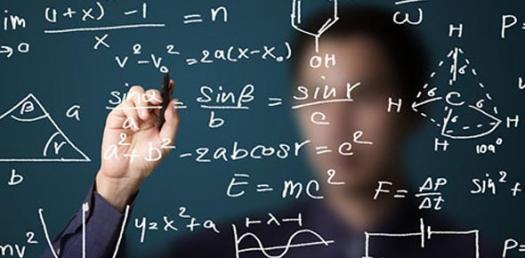# Chapter 13: Statistics Quiz

46 Questions | Total Attempts: 99SettingsThey may seem boring when you’re younger, but statistics are absolutely fascinating when you get down to the nitty-gritty of them. Today we’ll be doing just that by testing your knowledge on statistics, what kinds of data it deals with and what it’s used for. Good luck!

• 1.
The art and science of gathering, analyzing, and making inferences (predictions) from numerical information obtained in an experiment
• A.

Population

• B.

Statistics

• C.

cluster sample

• D.

Class mark

• 2.
As the numerical information so obtained is referred
• A.

unbiased sample

• B.

area sample

• C.

frequency distribution

• D.

Data

• 3.
Is concerned with the collection, organization, and analysis of data
• A.

Descriptive statistics

• B.

Inferential statistics

• C.

random number generator

• D.

piece of data

• 4.
Is concerned with making generalizations or predictions from the data collected
• A.

Descriptive statistics

• B.

Inferential statistics

• C.

random number generator

• D.

Piece of data

• 5.
The entire contents of the box constitute the population, it consists of all items or people of interest
• A.

Population

• B.

area sample

• C.

Frequency distribution

• D.

midpoint of a class

• 6.
It’s the statistician often uses a subset of the population
• A.

Statistics

• B.

random number table

• C.

Sample

• D.

convenience sample

• 7.
The sum of the data divided by the number of pieces of data,it  is used when the mean of a sample of the population is calculated
• A.

s

• B.

µ

• C.

¯x (x bar)

• D.

σ

• 8.
Standard deviation, measures how much the data differ from the mean. It is used when the standard deviation by of a sample is calculated
• A.

σ

• B.

S

• C.

µ

• D.

¯x (x bar)

• 9.
The sum of the data divided by the number of pieces of data, it is used when the mean of a sample of the entire population is calculated
• A.

¯x (x bar)

• B.

µ

• C.

σ

• D.

S

• 10.
Standard deviation, measures how much the data differ from the mean, it is used when the standard deviation of the entire population is calculated
• A.

¯x (x bar)

• B.

µ

• C.

σ

• D.

S

• 11.
Is one that is a religion, political affiliation, age, and so on…
• A.

Systematic sample

• B.

Frequency distribution

• C.

convenience sample

• D.

Unbiased sample

• 12.
If a sample is drawn in such a way that each time an item is selected each item in the population has an equal chance of being drawn.
• A.

random sample

• B.

Cluster sample

• C.

systematic sample

• D.

convenience sample

• 13.
When a sample is obtained by drawing every nth item on a list or production line.
• A.

convenience sample

• B.

Random sample

• C.

systematic sample

• D.

cluster sample

• 14.
Is sometimes referred to as an area sample because it is frequently applied on a geographical basis
• A.

convenience sample

• B.

Random sample

• C.

systematic sample

• D.

cluster sample

• 15.
It uses data that are easily or readily obtained
• A.

convenience sample

• B.

Random sample

• C.

systematic sample

• D.

cluster sample

• 16.
It is a device, usually a calculator or computer program, that produces a list of random number
• A.

random number table

• B.

Random number generator

• C.

area sample

• 17.
It is a collection of random digits in which each digits has an equal chance or appearing
• A.

random number table

• B.

Random number generator

• C.

area sample

• 18.
When a population is divided into parts, called strata, for the  purpose of drawing a sample
• A.

unbiased sample

• B.

piece of data

• C.

stratified sampling

• D.

class width

• 19.
Known as class, when a population has varied characteristics and then take  a random sample from each of class.
• A.

stratum

• B.

Piece of data

• C.

Descriptive statistics

• D.

Population

• 20.
It is a single response to an experiment. When the amount of date is large, it is usually advantageous to construct a frequency distribution
• A.

Piece of data

• B.

class width

• C.

class mark

• D.

histogram

• 21.
A listing of the observed values and the corresponding  frequency of occurrence of each value
• A.

stem-and-leaf display

• B.

frequency polygon

• C.

frequency distribution

• D.

measures of central tendency

• 22.
To determine how far, in terms of standard deviations, a given score is from the mean of the distribution
• A.

z-score

• B.

rectangular distribution

• C.

Measures of dispersion

• D.

Measures of position

• 23.
It divided data into 4 equal parts, the 1st is the value that is higher than about ¼ or 25% of the population, the 2nd is the value that is higher than about ½ the population and is the same as the 50th percentile, or the median, the 3rd is the value that is higher than about ¾ of the population and is the same as the 75th percentile
• A.

median

• B.

measures of position

• C.

percentile

• D.

quartiles

• 24.
The mean, median, and mode all have the same value
• A.

skewed distribution

• B.

Gaussian distribution

• C.

J-shaped distribution

• D.

Rectangular distribution

• 25.
It has more of a “tail” on one side than the other
• A.

Gaussian distribution

• B.

skewed distribution

• C.

J-shaped distribution

• D.

Rectangular distribution

Related TopicsBack to top